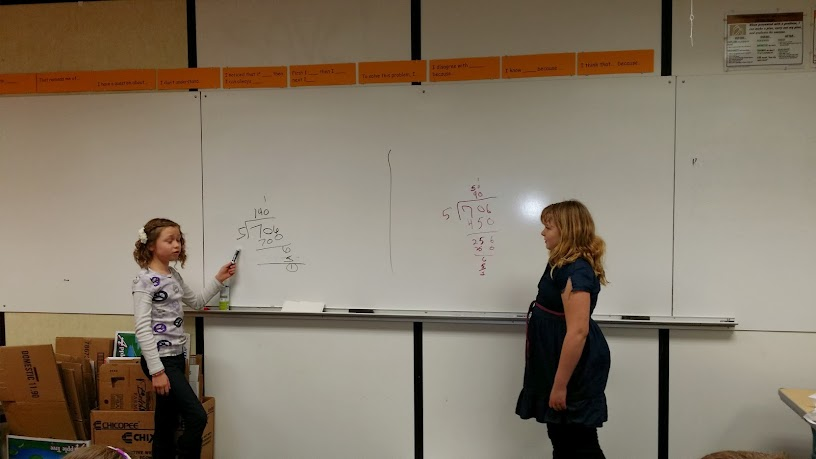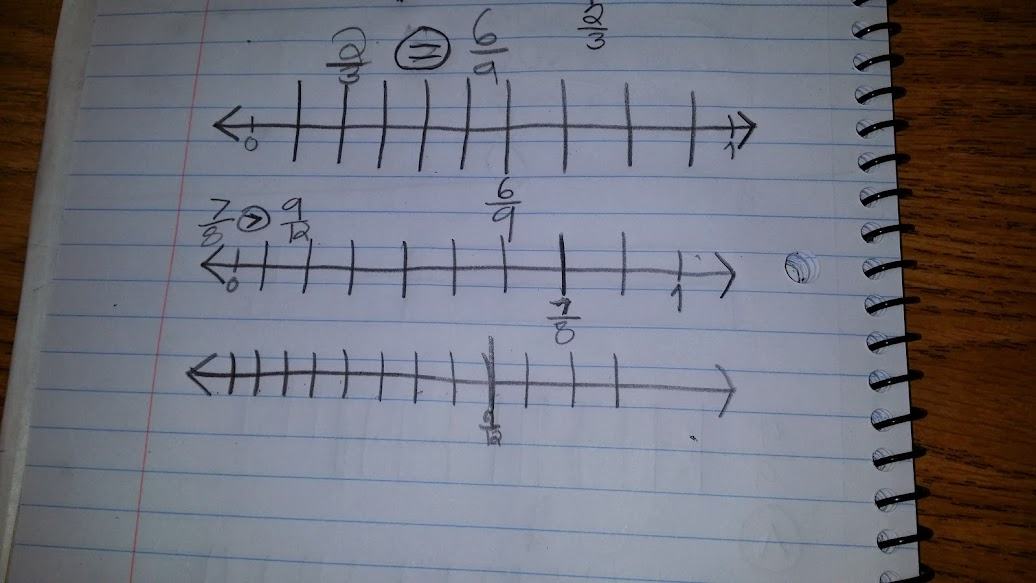# Comparing Fractions Using a Number Line

48 teachers like this lesson
Print Lesson

## Objective

SWBAT compare fractions with different denominators by using number lines.

#### Big Idea

This lesson builds towards students ability to compare fractions by creating common denominators or numerators - 4.NF.2

## Number Hook

7 minutes

Fr this number hook, I give students a riddle rather than a magic trick. I roll five dice and show the dice under the document camera.  Then I tell students, "The name of the game is Polar Bears Around the Ice Hole. The name of the game is important! How many polar bears are there?"

The following is an example of how this looked during this riddle:

The first roll produced 4, 6, 1, 3, 2. "Six," said Billy. "No, two," Johnny replied. The next roll was 5, 1, 5, 2, 4. "Four?" said Billy. "No, eight," Johnny said. The next rolls were 3, 5, 3, 1, 2. There were 8 polar bears. The next rolls were 6, 2, 1, 2, 4. There were no polar bears. How does Johnny figure out the number of polar bears?

The answer to this riddle is quite simple, but one that my students have not figured out yet. My students LOVE this riddle and based on previous experience, I predict I will get many requests to play this again, often.

Dice all look the same. On a die, the 1, 3, and 5 all have a dot in the center. The 3 has 2 dots on either side of the center dot, and the 5 has 4 dots around the center dot. Johnny simply counted the number of dots around the outside. A "3" has 2 "petals around the rose, or polar bears around an ice hole." The "5" has 4 "petals" or "polar bears." Roll some dice and it will become clear!!

## Warm Up

7 minutes

I chose to give my students a division problem for a warm up today. I want to ensure that my students continue to build their problem solving skills and make sense of problems in addition to keeping the skill of division strong. I put this problem on the board.

A movie store had 706 movies that they were putting onto 5 shelves.  If the owner wanted to make sure each shelf had the same amount, how many more movies would she need?

After most students are finished, I ask 2 student volunteers to come to the board and solve the problem or show how they solved the problem. I ask the students to tell the class how he/she knows their answer is correct as well.

In this photo, you can see two students that did the division very differently, but got the same quotient of 141 remainder 1.  These students had different answers for "how many more movies the owner would need." The student on the left thought the remainder was the answer, and the student on the right thought the answer was 4, which is the correct answer.  We were able to have a great discussion about making sense of this problem and really delve into Math Practice 1 by doing so. About 1/5 of my class is still struggling with making sense of problems that have remainders.## Concept Development

35 minutes

This lesson involves comparing fractions using a number line.  The Common Core stresses the importance of moving from concrete fractional models to the representation of fractions using numbers and the number line.  Concrete fractional models are an important initial component in developing the conceptual understanding of fractions. However, it is vital that I link these models to fraction numerals and representation on the number line.  This movement from visual models to fractional numerals should be a gradual process as my students gain understanding of the meaning of fractions.

This lesson is also important in developing students ability to compare fractions by using a benchmark fraction or creating same denominators. While this lesson does not have students create same denominators, it does build students conceptual understanding of equivalent fractions. Students will place fractions like 2/3 and 6/9 on a number line and be able to see that they represent the same amount or part of the whole. This understanding is important before students can be successful with creating same denominators to compare fractions.

I begin this lesson by playing a few rounds of this brainpop game on the smartboard for all students.  I like this game because it reinforces the concept that fractions are number and have a place on the number line.

Next I show this learnzillion video. One of the aspects I really like about this video, (and the learnzillion fraction video series) is the review of numerator and denominator at the beginning of each lesson. This is so important for my students who are still struggling with the meaning of numerator and denominator.

After viewing the video, I draw two number lines with end points 0 and 1 on the board for all students to see. I then write these two fractions; 4/9 and 7/11   I model how to draw the two number lines parallel to each other just like in the  learnzillion video. I divide the first number line into 9 equal parts.  I explain to students that while I am just eyeballing the equal parts, it is important to be as precise as I can in order for the number line model to be helpful when comparing numbers using a number line.  Next I divide the second number line into 11 equal parts. I ask students where I would place the 4/9 on the first number line and where to place the 7/11 on the second number line.  I ask a student volunteer to come to the board to write the placement of each fraction. Once each fraction is placed on the number line, I model writing the number sentence 4/9 < 7/11.  Note: One strategy I use in order for students to have equal and equally spaced parts is to encourage students to draw long number lines.  It is easier for students to divide two long strips of paper into 9ths and 11ths and determine the part size equally versus if the number line is short

This is an example of a student's work showing fractions on a number line.Students draw 10 number lines on a piece of paper. I then give them 10 different fraction pairs to compare. Students model the fractions on parallel number lines. As students work, I circulate the room to observe students strategies and thinking.

## Wrap Up - Student Debrief

10 minutes

To conclude this lesson, I lead a discussion about using tools strategically and that number lines are not ALWAYS the best way to compare fractions.  I give students these two fractions to compare; 5/6 and 6/7

Students quickly realize that both fractions are one part away from being one whole.  Many of my students were able to think about the size of the parts and conclude that 6/7 is larger because it's parts are small, therefore the part away from one whole is also smaller than the part in 5/6.

This is still a very difficult concept for my students to think about and one I will continue to talk about often in future lessons.